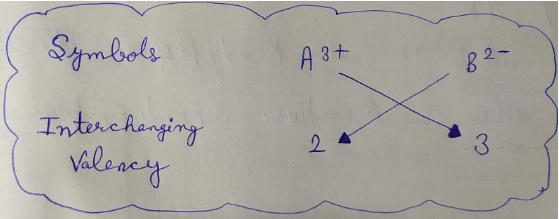Courses

# Test: Atomic And Molecular Mass

## 10 Questions MCQ Test Science Class 9 | Test: Atomic And Molecular Mass

Description
This mock test of Test: Atomic And Molecular Mass for Class 9 helps you for every Class 9 entrance exam. This contains 10 Multiple Choice Questions for Class 9 Test: Atomic And Molecular Mass (mcq) to study with solutions a complete question bank. The solved questions answers in this Test: Atomic And Molecular Mass quiz give you a good mix of easy questions and tough questions. Class 9 students definitely take this Test: Atomic And Molecular Mass exercise for a better result in the exam. You can find other Test: Atomic And Molecular Mass extra questions, long questions & short questions for Class 9 on EduRev as well by searching above.
QUESTION: 1

### Which of the following is correct pair of elements and its symbol?

Solution:

As we know, in the periodic table;
The symbol S  is used for sulphur
The symbol Ag is used for silver
The symbol Na is used for sodium
The symbol K  is used for potassium
So, option ( d) is the correct✔ answer.

QUESTION: 2

### Valency of hydrogen is 1 and that of sulphur is 2. What should be the formula of hydrogen sulphide?​

Solution:

Hydrogen sulfide is the chemical compound with the chemical formula H2S. It is a colorless gas with the characteristic foul odor of rotten eggs. It is very poisonous, corrosive, and flammable.

QUESTION: 3

### One atomic mass unit is a mass unit equal to exactly one twelfth (1/12th) the mass of one atom of ___________.

Solution:

An atomic mass unit is defined as a mass equal to one twelfth the mass of an atom of carbon 12. The mass of any isotope of any element is expressed in relation to the carbon 12 standard.

QUESTION: 4

Which of the following is the correct IUPAC symbol for the element Cobalt?

Solution:
The wrong thing in writing cobalt as CO is that we can't write o as O because O represent Oxygen and symbols representing an element with two letters have first one as capital and next one should be in small letter.
Therefore we should always write cobalt as..Co not CO!

QUESTION: 5

Which one of the following is the modern symbol of Gold?

Solution: Gold is a chemical element with symbol Au (from Latin: aurum) and atomic number 79, making it one of the higher atomic number elements that occur naturally. In its purest form, it is a bright, slightly reddish yellow, dense, soft, malleable, and ductile metal.
QUESTION: 6

An atom has a mass number of 23 and atomic number 11. The number of protons are ________.

Solution:

A neutral atom will have the samenumber of electrons is does protons. Since it has an atomic number of 11 it must have 11 protons. Since it has anatomic mass of 23 it must have 12 neutrons.

QUESTION: 7

NaCl molecule is made of which of the following ions?

Solution: An oxygen molecule (O2) is a good example of a molecule with a covalent bond. Ionic bonds occur when electrons are donated from one atom to another. Table salt (NaCl) is a common example of a compound with an ionic bond.
QUESTION: 8

Valency of Calcium element is:

Solution:

The valency of Calcium of 2+. Calcium has 2 electrons in its outermost shell, its electronic configuration being (2,8,8,2). In order to attain stability it loses 2 electrons to become stable according to the octet rule.

QUESTION: 9

Chemical formula of a compound is A2B3. The valency of A is

Solution:QUESTION: 10

What is the atomic mass of nitrogen?

Solution:
Atomic Mass is determined by total number of nucleons in nucleus. Total number of nucleons is equal to sum of proton and neutrons in a atom .So, number of protons in Nitrogen is 7 as well as Neutron present are also 7. As 7 +7 is 14, the atomic mass is 14 u,(small u) is the atomic mass unit .